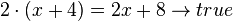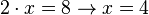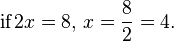# Equation facts for kids

Kids Encyclopedia Facts

A mathematical equation is an expression containing at least one variable (=unknown value) and an "equals sign" ( = ) with a mathematical expression on each side of it. The equals sign says that both sides are exactly the same value. An equation can be as simple as x=0, or as hard as 4(3y^99)+76=42÷3x or harder.

There are two kinds of mathematical equations:

• The kind of equation that is either true or false; these are also called identities. For example:$2 \cdot (x+4)=2x+8 \rightarrow true$
• The kind of equation that lets you calculate the value of one or several variables. The equation is only true if the variable(s) have that value. For example:$2 \cdot x=8 \rightarrow x=4$

The second kind is often used to solve problems in which you have to know the value of some variables. For example,$\text{if}\, 2x = 8, \, x = \frac{8}{2} = 4.$

The second kind of equation is used in algebra. For example, to solve the equation 2x = 8 by finding x you would follow an algebraic rule. Then you can work out that x = 4.

## Types of equations

Equations can be classified by the types of operations and quantities involved. Some types of equations are:

## Images for kidsEquation Facts for Kids. Kiddle Encyclopedia.